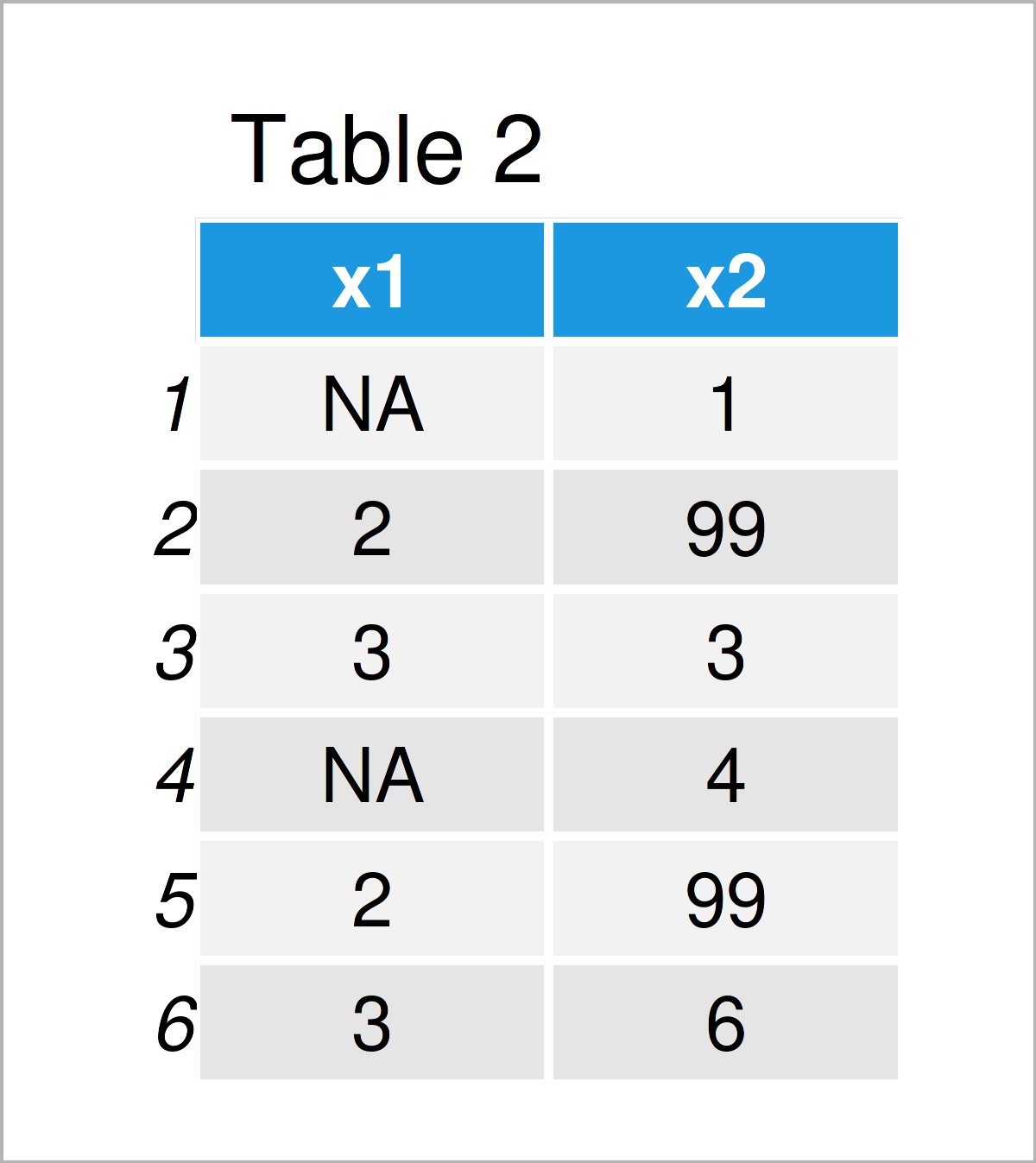# R Error missing values are not allowed (2 Examples)

In this article, I’ll demonstrate how to deal with the “Error in X : missing values are not allowed in subscripted assignments of data frames” in R programming.

The page is structured as follows:

So without further additions, let’s dive right in!

## Creation of Example Data

The following data will be used as basement for this R tutorial:

```data <- data.frame(x1 = c(NA, 2, 3, NA, 2, 3),     # Create example data
x2 = 1:6)
data                                               # Print example data```As you can see based on Table 1, the exemplifying data is a data frame comprising six rows and two columns called “x1” and “x2”. The variable x1 has the numeric class and the variable x2 has the integer class. Note that some of the values in our data are missing (i.e. NA).

## Example 1: Reproduce the Error – missing values are not allowed in subscripted assignments of data frames

The following R code demonstrates how to replicate the “Error in X : missing values are not allowed in subscripted assignments of data frames”.

Let’s assume that we want to replace values in the variable x2 conditionally based on the variable x1. Then, we might try to use the following R code:

```data[data\$x1 == 2, ]\$x2 <- 99                      # Try to replace values
# Error in `[<-.data.frame`(`*tmp*`, data\$x1 == 2, , value = list(x1 = c(NA,  :
#   missing values are not allowed in subscripted assignments of data frames```

Unfortunately, the previous R code returns the error message “Error in X : missing values are not allowed in subscripted assignments of data frames”.

The reason for this is that the variable x1 contains NA values.

In the next example, I’ll show how to fix this problem!

## Example 2: Fix the Error – missing values are not allowed in subscripted assignments of data frames

Example 2 demonstrates how to avoid the “Error in X : missing values are not allowed in subscripted assignments of data frames”.

To do this, we can use the is.na function in our logical condition. Consider the following R code:

```data[data\$x1 == 2 & !is.na(data\$x1), ]\$x2 <- 99    # Properly replace values
data                                               # Print updated data```Table 2 illustrates the output of the previous syntax: A data frame with substituted values.

## Video & Further Resources

Would you like to learn more about the dealing with the “Error in X : missing values are not allowed in subscripted assignments of data frames”? Then you might watch the following video on my YouTube channel. I show the R syntax of this tutorial in the video:

Also, you might have a look at the other tutorials on this homepage.

In this R tutorial you have learned how to debug the “Error in X : missing values are not allowed in subscripted assignments of data frames”.

Note that a very similar error message, i.e. “NAs are not allowed in subscripted assignments”, can occur when dealing with vector objects instead of data frame columns. In case of this error, make sure to include the is.na function in your logical condition.